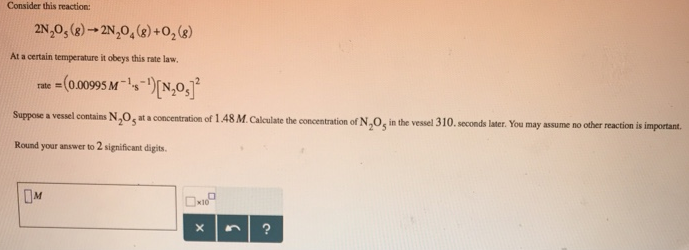# Problem: Consider this reaction:2N2O5 (g) → 2N2O4 (g) + O2 (g) At a certain temperature it obeys this rate law. rate = (0.00995 M-1 • s^-1)[N2O5]2 Suppose a vessel contains N2O5 at a concentration of 1.48 M. Calculate the concentration of N2O5 in the vessel 310. seconds later. You may assume no other reaction is important. Round your answer to 2 significant digits.

###### FREE Expert Solution
82% (219 ratings)###### Problem Details

Consider this reaction:

2N2O5 (g) → 2N2O(g) + O2 (g)

At a certain temperature it obeys this rate law.

rate = (0.00995 M-1 • s^-1)[N2O5]

Suppose a vessel contains N2O5 at a concentration of 1.48 M. Calculate the concentration of N2O5 in the vessel 310. seconds later. You may assume no other reaction is important.

Round your answer to 2 significant digits.Frequently Asked Questions

What scientific concept do you need to know in order to solve this problem?

Our tutors have indicated that to solve this problem you will need to apply the Integrated Rate Law concept. You can view video lessons to learn Integrated Rate Law. Or if you need more Integrated Rate Law practice, you can also practice Integrated Rate Law practice problems.

What professor is this problem relevant for?

Based on our data, we think this problem is relevant for Professor Stein's class at UCONN.